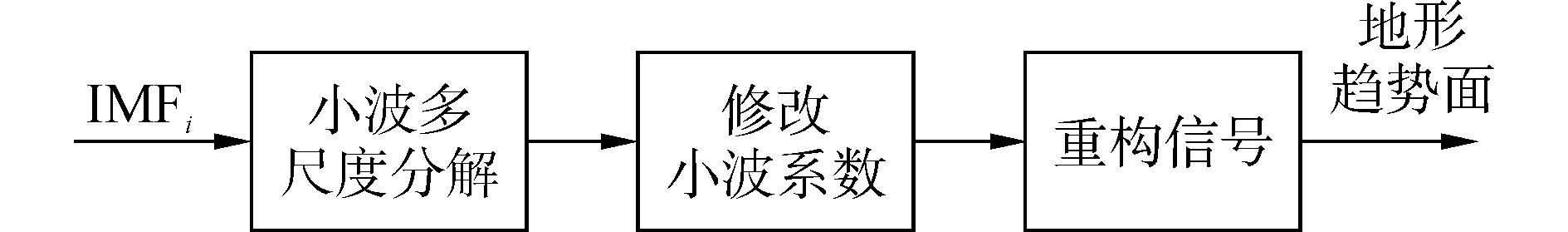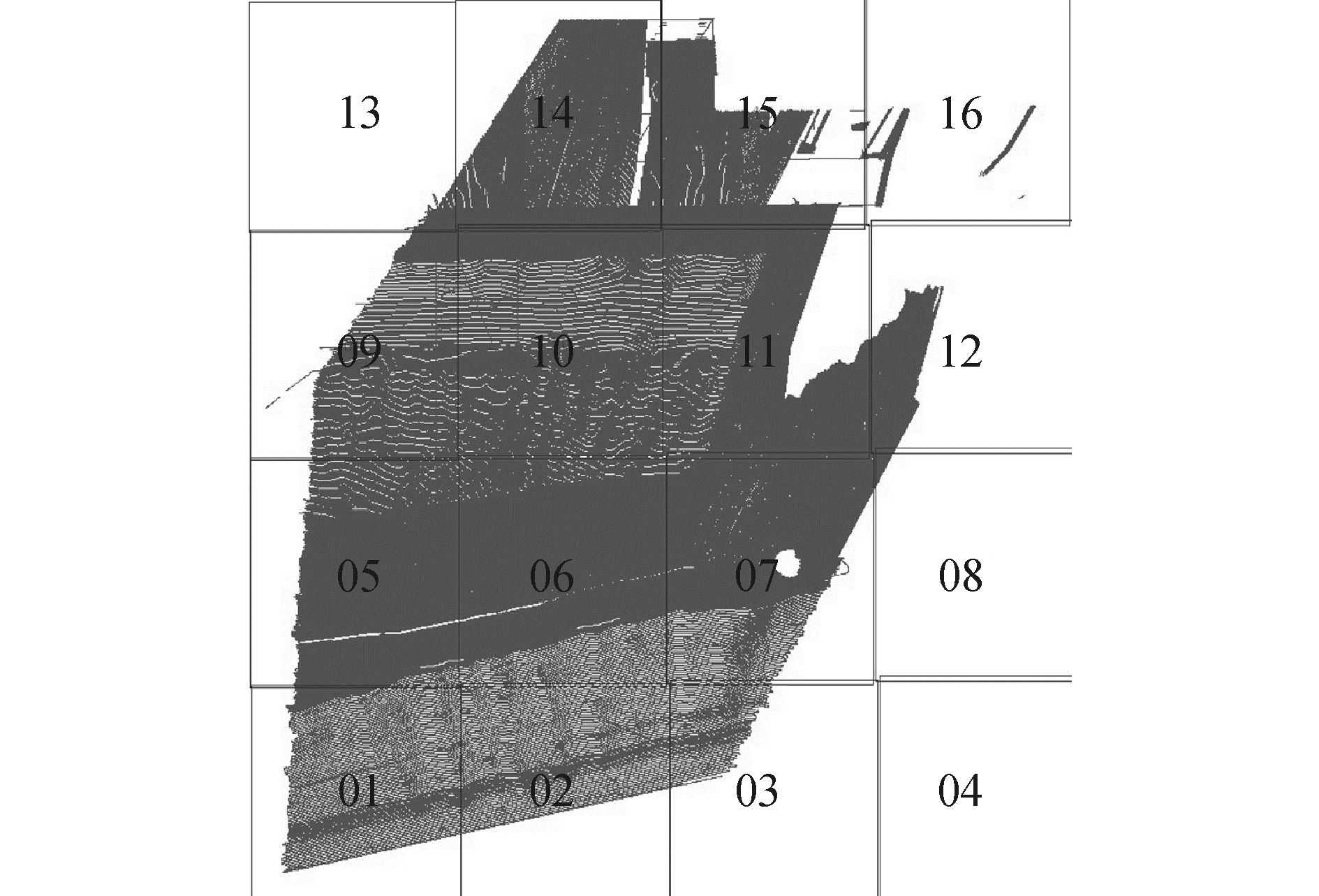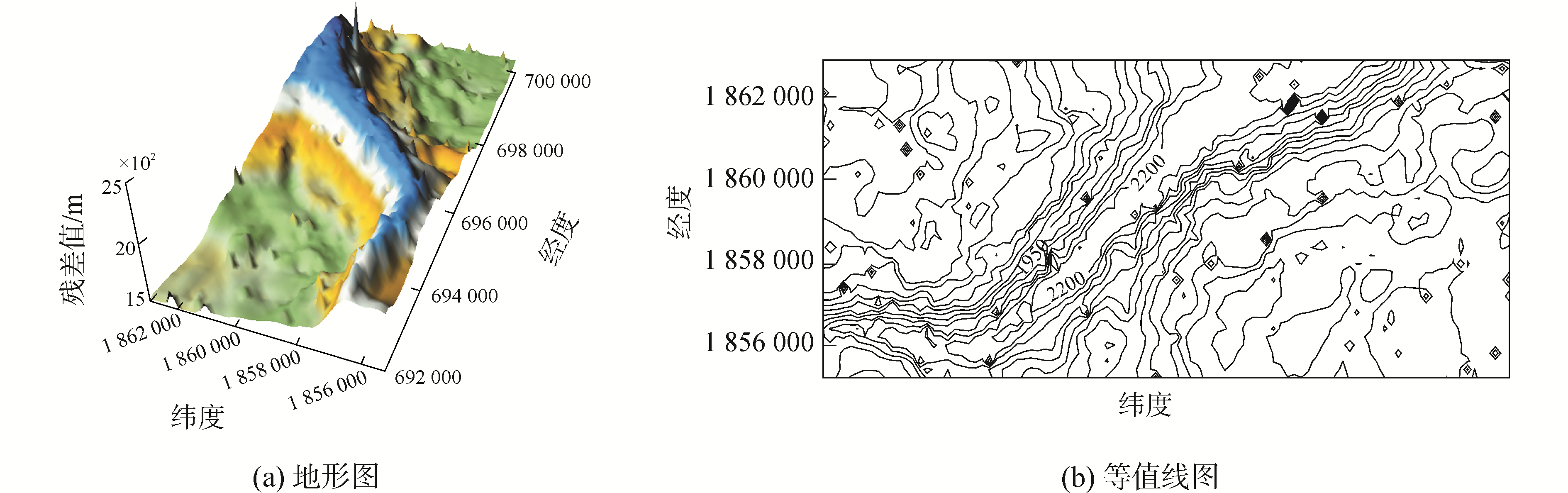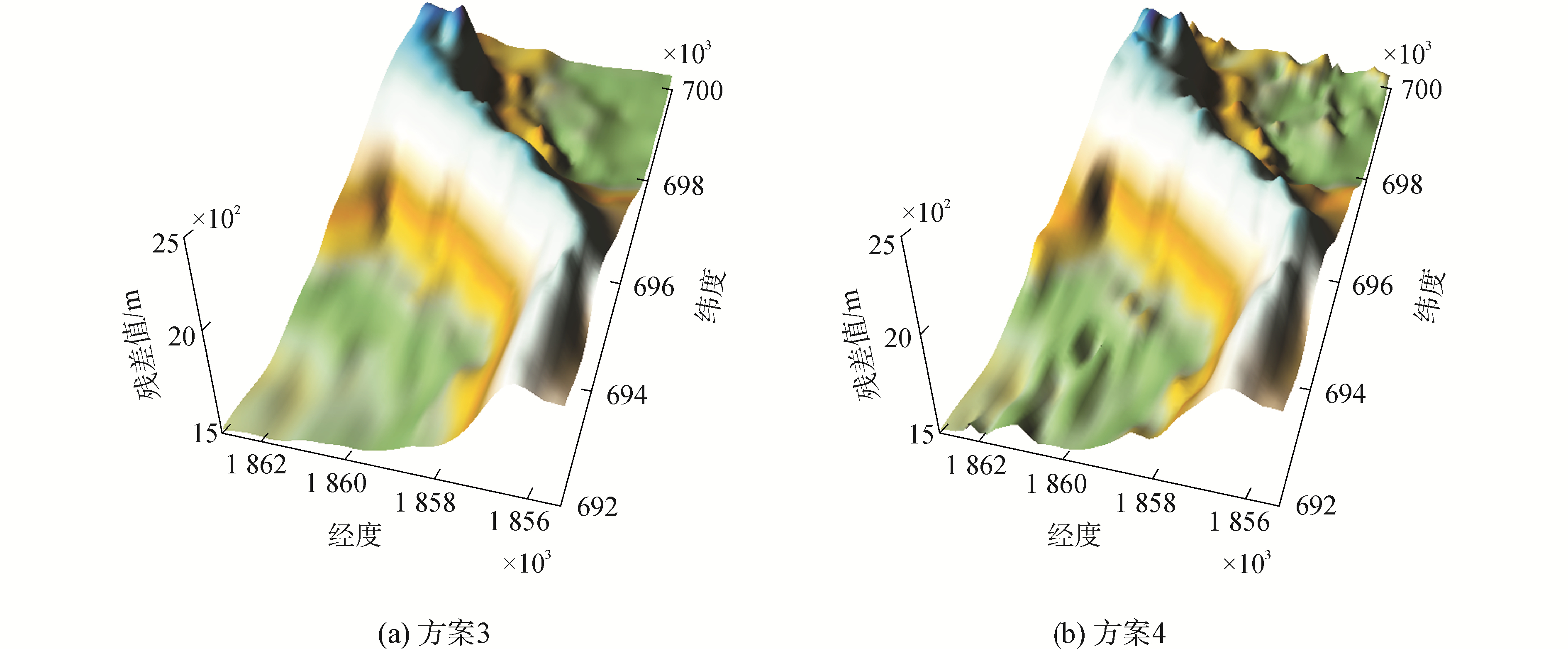﻿ 多波束测深异常数据探测方法
«上一篇文章快速检索 高级检索

 哈尔滨工程大学学报2018, Vol. 39Issue (9): 1458-1464  DOI: 10.11990/jheu.2017040590

### 引用本文ZHAO Xianghong, BAO Jingyang. Method for detecting outliers of multi-beam sounding data[J]. Journal of Harbin Engineering University, 2018, 39(9), 1458-1464. DOI: 10.11990/jheu.201704059.### 文章历史

Method for detecting outliers of multi-beam sounding data
ZHAO Xianghong, BAO Jingyang
Department of Hydrography and Cartography, Dalian Naval Academy, Dalian 116018, China
Abstract: In view of the difficulty of detecting abnormal data under the condition of complex seabed terrain, a method for detecting the outliers of multi-beam bathymetric data was proposed on basis of BEMD method. The original BEMD algorithm was improved, the neighboring window method was used to extract the local extreme point and the radial basis function interpolation method was used to fit the upper and lower envelope. Wavelet analysis was carried out for the eigenfunction IMF obtained by BEMD method, trying to strip away noise and useful signal; useful signal was reconstituted, in addition, in combination with the residual component obtained by empirical mode decomposition, the sounding data outliers of the seabed terrain trend face were constituted. By comparing with manual editing method, trend surface filtering method and single BEMD, it was verified that the method is reasonable and effective for detecting outliers.
Keywords: multi-beam    radial basis functions interpolation    bidimensional empirical mode decomposition    trend surface filtering    outliers    wavelet analysis

Huang等提出一种新的数据处理方法，即经验模态分解法，该方法能够保留数据本身的特点，将信号中不同尺度的波动或趋势逐级分解开来，产生一系列本征模态函数。关于经验模态分解方法的研究，专家们做了大量的工作[9-14]，但是该方法在海洋测深数据处理方面的应用较少。

1 二维经验模态方法 1.1 方法原理

1) 提取原始信号f(x, y)的极大值和极小值点，并对其进行插值拟合计算上、下包络面max(x, y)和min(x, y)；计算上、下包络面均值m(x, y)=[min(x, y)+max(x, y)]/2。

2) 原始数据f(x, y)减去计算得到的包络面均值得到H1(x, y)=f-m。判断H1(x, y)是否为本征模态函数，若是则作为第一阶本征模态函数，若不是则令f(x, y)=H1(x, y)，重复前步过程直至满足条件。最终得到第一阶本征模态函数：IMF1=H1(x, y)。

3) 将得到的IMF1从原始信号中分离出去，得到残余量R1=f(x, y)-IMF1，将R1作为新的数据，重复循环上述过程，直至满足筛分终止条件

1.2 关键问题研究 1.2.1 局部极值点提取

 $\mathit{\boldsymbol{X}} = \left[ {\begin{array}{*{20}{c}} {{x_{11}}}&{{x_{12}}}& \cdots &{{x_{1n}}}\\ {{x_{21}}}&{{x_{22}}}& \cdots &{{x_{2n}}}\\ \cdots&\cdots&\cdots&\cdots \\ {{x_{m1}}}&{{x_{m2}}}&{}&{{x_{mn}}} \end{array}} \right]$ (1)

 ${x_{ij}} = \left\{ {\begin{array}{*{20}{c}} \begin{array}{l} \max \left( {x,y} \right)\\ \min \left( {x,y} \right) \end{array}&\begin{array}{l} {x_{ij}} \ge {x_{pq}}\\ {x_{ij}} < {x_{pq}} \end{array} \end{array}} \right.,$

1.2.2 包络面插值方法

 $S\left( x \right) = {p_m}\left( x \right) + \sum\limits_{i = 1}^N {{\lambda _i}\phi \left( {\left\| {x - {x_i}} \right\|} \right)}$ (2)表 1 径向基函数的不同核函数及表达式 Tab.1 Different kernel functions of radial basis interpolation and its expression表 2 几种核函数对径向基插值结果的比较 Tab.2 Comparison results of several kernel functions of radial basis interpolationm

 $\left[ {\begin{array}{*{20}{c}} \mathit{\boldsymbol{B}}&\mathit{\boldsymbol{P}}\\ {{\mathit{\boldsymbol{P}}^{\rm{T}}}}&{\bf{0}} \end{array}} \right]\left[ {\begin{array}{*{20}{c}} \mathit{\boldsymbol{\lambda }}\\ \mathit{\boldsymbol{C}} \end{array}} \right] = \left[ {\begin{array}{*{20}{c}} \mathit{\boldsymbol{H}}\\ {\bf{0}} \end{array}} \right]$ (3)

bij表示不同核函数对应系数，$\mathit{\boldsymbol{P}} = \left[ {\begin{array}{*{20}{c}} 1&{{x_1}}&{{y_1}}\\ \cdots&\cdots&\cdots \\ 1&{{x_n}}&{{y_n}} \end{array}} \right]$λ=[λ1  λ2  …  λn]，C=[C0  C1  C2]，H=[H1  H2  …  Hn]T，式(3)中只有λC是未知的，可以通过带入样本计算得到λ0C0，最终插值结果如下

 $\left[ {\begin{array}{*{20}{c}} \mathit{\boldsymbol{H}}\\ {\bf{0}} \end{array}} \right] = \left[ {\begin{array}{*{20}{c}} \mathit{\boldsymbol{A}}&\mathit{\boldsymbol{P}}\\ {{\mathit{\boldsymbol{P}}^{\rm{T}}}}&{\bf{0}} \end{array}} \right]\left[ {\begin{array}{*{20}{c}} {{\mathit{\boldsymbol{\lambda }}_0}}\\ {{\mathit{\boldsymbol{C}}_0}} \end{array}} \right]$ (4)Download: 图 1 包络面插值方法比较 Fig. 1 Comparison of interpolation method of envelope surface表 3 不同插值方法插值精度比较 Tab.3 Comparison of interpolation precision of several interpolation methodsmDownload: 图 2 差值三维图 Fig. 2 Three-dimensional diagram of difference value
1.2.3 趋势面构造及异常值探测原理

 $D\left( t \right) = \sum\limits_{i = 1}^n {{\rm{IM}}{{\rm{F}}_i}} + R$ (5)Download: 图 3 小波变换提取信号 Fig. 3 Signal extracted by wavelet transform

 ${W_f}\left( {a,b} \right) = < f,{\psi _{a,b}}\left( t \right) \ge {\left| a \right|^{ - \frac{1}{2}}}\int_{ - \infty }^{ + \infty } {f\left( t \right)\bar \psi \left( {\frac{{t - b}}{a}} \right){\rm{d}}t}$ (6)
 ${\psi _{a,b}}\left( t \right) = {\left| a \right|^{ - \frac{1}{2}}}\psi \left( {\frac{{t - b}}{a}} \right)$ (7)

 $F' = \left\{ {\begin{array}{*{20}{c}} \begin{array}{l} {\rm{sign}}\left( F \right)\left( {\left| F \right| - t} \right),\\ 0, \end{array}&\begin{array}{l} \left| F \right| \ge t\\ \left| F \right| < t \end{array} \end{array}} \right.$ (8)

 $\left\{ \begin{array}{l} {Z_i} \le k\sigma ,k = 2\;或\;3,第\;i\;个水深点为正常\\ {Z_i} > k\sigma ,k = 2\;或\;3,第\;i\;个水深点为异常值 \end{array} \right.$ (9)
2 实验验证与分析Download: 图 4 测区多波束测深条带布设 Fig. 4 Measure area of mult-beam strip layoutDownload: 图 5 原始含异常值的水深数据 Fig. 5 Original water depth data containing outliersDownload: 图 6 基于Surfer 8.0软件显示的海底地形图 Fig. 6 Seabed topographic map based on Surfer 8.0Download: 图 7 方案3与方案4构建的海底地形趋势面比较 Fig. 7 Comparison of seabed contour map of plan 3 and plan 4表 4 几种方案数据剔除效果比较 Tab.4 Statics of comparison of several plans gross error elimination
3 结论

1) 虽然水深数据中的异常值出现约仅占整体数据的1%~5%，但是在精确海底地形，确保航行安全的过程中，异常值的影响不容忽视，必须进行剔除工作。

2) 趋势面滤波法采用多项式拟合海底地形进行相应水深点的精度评定，其优点是算法简单易于编程实现，并且当海底地形较为品平缓的情况下该方法的异常值探测效果较好；其缺点是当海底地形较为复杂的情况下，异常值剔除效果较差且会剔除大量真实水深数据导致地形被人为改变。

3) 仅依靠二维经验模态分解的异常值剔除方法，其优点是可以依据数据本身特点，构建海底地形趋势面并探测出异常数据；其缺点是海底地形趋势面是由分解得到的残余量构建，仅包含原始数据中低频成分，不能精确反映海底地形特点，仍然存在部分未能探测到的异常数据，需要结合其他方法或者考虑分块处理。

4) 二维经验模态分解产生的本征模态函数中包含信号细节信息，经过小波分析方法可以提取出其有用信号，经过有用信号与经验模态分解得到的残余量构建的海底地形趋势面可精确反应海底真实情况，其异常值剔除效果好于单一经验模态分解方法。

  李家彪. 多波束勘测原理技术与方法[M]. 北京: 海洋出版社, 1999. (0)  赵建虎, 刘经南. 多波束测深及图像数据处理[M]. 武汉: 武汉大学出版社, 2008. (0)  黄谟涛, 翟国君, 王瑞, 等. 海洋测量异常数据的检测[J]. 测绘学报, 1999, 28(3): 269-277. HUANG Motao, ZHAI Guojun, WANG Rui, et al. The Detection of abnormal data in marine survey[J]. Acta geodaetica et cartographica sinica, 1999, 28(3): 269-277. DOI:10.3321/j.issn:1001-1595.1999.03.015 (0)  黄贤源, 翟国君, 隋立芬, 等. LS-SVM算法中优化训练样本对测深异常值剔除的影响[J]. 测绘学报, 2011, 40(1): 22-27. HUANG Xianyuan, ZHAI Guojun, SUI Lifen, et al. The Influence of optimized train samples on elimination of sounding outliers in the LS-SVM arithmetic[J]. Acta geodaetica et cartographica sinica, 2011, 40(1): 22-27. (0)  黄辰虎, 陆秀平, 侯世喜, 等. 利用CUBE算法剔除多波束测深粗差研究[J]. 海洋测绘, 2010, 30(3): 1-5. HUANG Chenhu, LU Xiuping, HOU Shixi, et al. Study on detecting outlier of multibeam sounding based on CUBE algorithm[J]. Hydrographic surveying and charting, 2010, 30(3): 1-5. DOI:10.3969/j.issn.1671-3044.2010.03.001 (0)  阳凡林, 刘经南, 赵建虎. 多波束测深数据的异常检测和滤波[J]. 武汉大学学报(信息科学版), 2004, 29(1): 80-83. YANG Fanlin, LIU Jingnan, ZHAO Jianhu. Detecting outliers and filtering noises in multi-beam data[J]. Geomatics and Information Science of Wuhan University, 2004, 29(1): 80-83. (0)  董江, 任立生. 基于趋势面的多波束测深数据滤波方法[J]. 海洋测绘, 2007, 27(6): 25-28. DONG Jiang, REN Lisheng. Filter of MBS sounding data based on trend surface[J]. Hydrographic surveying and charting, 2007, 27(6): 25-28. DOI:10.3969/j.issn.1671-3044.2007.06.007 (0)  HUANG N E, SHEN Zheng, LONG S R, et al. The empirical mode decomposition and the hilbert spectrum for nonlinear and non-stationary time series analysis[J]. Proceedings of the royal society A:mathematical, physical and engineering sciences, 1998, 454(1971): 903-995. DOI:10.1098/rspa.1998.0193 (0)  NUNES J C, BOUAOUNE Y, DELECHELLE E, et al. Image analysis by bidimensional empirical mode decomposition[J]. Image and vision computing, 2003, 21(12): 1019-1026. (0)  陈建国, 肖凡, 常韬. 基于二维经验模态分解的重磁异常分离[J]. 地球科学——中国地质大学学报, 2011, 36(2): 327-335. CHEN Jianguo, XIAO Fan, CHANG Tao. Gravity and magnetic anomaly separation based on bidimensional empirical mode decomposition[J]. (Earth science)——Journal of China University of Geosciences, 2011, 36(2): 327-335. (0)  张彦铎, 汪敏敏, 鲁统伟. 改进的二维经验模式分解方法[J]. 武汉工程大学学报, 2013, 35(4): 61-65. ZHANG Yanduo, WANG Minmin, LU Tongwei. Decomposition method of improved two-dimensional empirical mode[J]. Journal of Wuhan Institute of Technology, 2013, 35(4): 61-65. DOI:10.3969/j.issn.1674-2869.2013.04.014 (0)  李翠芸, 曹潇男, 姬红兵, 等. 基于偏微分方程的快速二维经验模态分解方法及其应用[J]. 计算机辅助设计与图形学学报, 2014, 26(7): 1143-1150, 1158. LI Cuiyun, CAO Xiaonan, JI Hongbing, et al. A Fast Bidimensional empirical mode decomposition based on partial differential equation and its application on image processing[J]. Journal of computer-aided design & computer graphics, 2014, 26(7): 1143-1150, 1158. (0)  舒忠平, 杨智春. 抑制经验模分解边缘效应的极值点对称延拓法[J]. 西北工业大学学报, 2006, 24(5): 639-643. SHU Zhongping, YANG Zhichun. A better method for effectively suppressing end effect of empirical mode decomposition (EMD)[J]. Journal of Northwestern Polytechnical University, 2006, 24(5): 639-643. DOI:10.3969/j.issn.1000-2758.2006.05.023 (0)  刘慧婷, 张旻, 程家兴. 基于多项式拟合算法的EMD端点问题的处理[J]. 计算机工程与应用, 2004, 40(16): 84-86, 100. LIU Huiting, ZHANG Min, CHENG Jiaxing. Dealing with the end issue of EMD based on polynomial fitting algorithm[J]. Computer engineering and applications, 2004, 40(16): 84-86, 100. DOI:10.3321/j.issn:1002-8331.2004.16.028 (0)